# Ch 9 Notes Chemical Quantities Stoichiometry refers to

• Slides: 14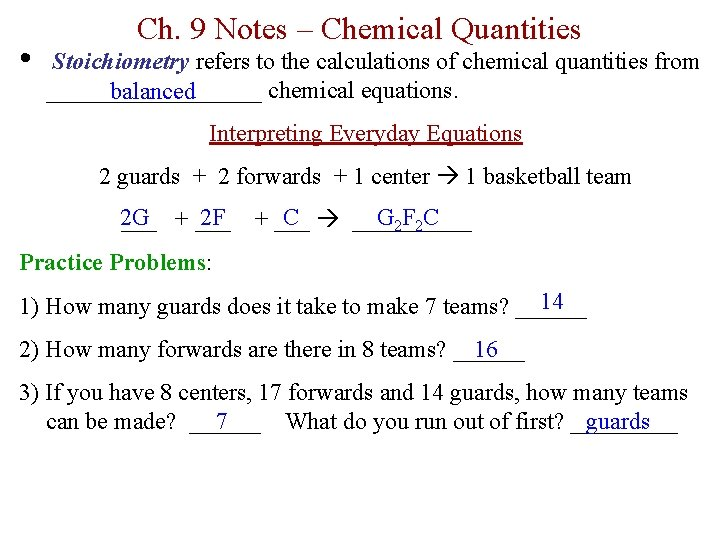• Ch. 9 Notes – Chemical Quantities Stoichiometry refers to the calculations of chemical quantities from _________ chemical equations. balanced Interpreting Everyday Equations 2 guards + 2 forwards + 1 center 1 basketball team 2 G 2 F ___ + ___ C _____ G 2 F 2 C + ___ Practice Problems: 14 1) How many guards does it take to make 7 teams? ______ 2) How many forwards are there in 8 teams? ______ 16 3) If you have 8 centers, 17 forwards and 14 guards, how many teams can be made? ______ What do you run out of first? _____ 7 guards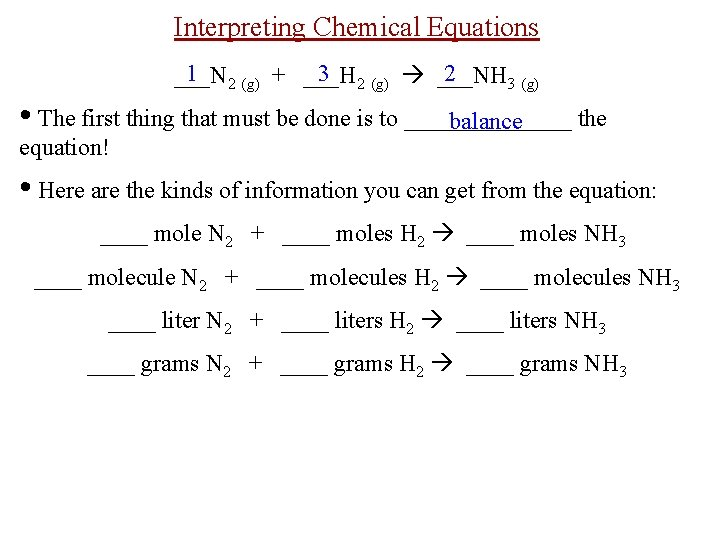Interpreting Chemical Equations 1 2 (g) + ___H 3 2 (g) ___NH 2 ___N 3 (g) • The first thing that must be done is to _______ the balance equation! • Here are the kinds of information you can get from the equation: ____ mole N 2 + ____ moles H 2 ____ moles NH 3 ____ molecule N 2 + ____ molecules H 2 ____ molecules NH 3 ____ liter N 2 + ____ liters H 2 ____ liters NH 3 ____ grams N 2 + ____ grams H 2 ____ grams NH 3Mole-Mole Conversions • coefficients The mole conversion factor comes from the _________ of the balanced chemical equation. Step 1: Write down the “given”. Step 2: Set up a conversion factor to change from moles to moles. Practice Problems: N 2 (g) + 3 H 2 (g) 2 NH 3 (g) 1) How many moles of ammonia can be made from 7 moles of nitrogen reacting with an excess of hydrogen? 7 mol N 2 x 2 mol NH 3 = 1 mol N 2 2) How many moles of hydrogen are required to completely react with 8 moles of nitrogen to produce ammonia? 8 mol N 2 x 3 mol H 2 = 1 mol N 2 3) How many moles of hydrogen are needed to react with an excess of nitrogen to make 10 moles of ammonia? 3 mol H 2 = 10 mol NH 3 x 2 mol NH 3Other Conversion Problems • Mass-Mass: (grams to moles to grams) Step 1: Write down the “given” and convert from grams to moles. Step 2: Convert from moles of the “given” to moles of the “unknown” using a mole conversion factor. Step 3: Convert from moles of the unknown to grams. N 2 (g) + 3 H 2 (g) 2 NH 3 (g) Practice Problem: How many grams of ammonia can be made from reacting 39. 0 grams of nitrogen with an excess of hydrogen? 39. 0 g N 2 x x x =Other Conversion Problems • Volume-Mass: (liters to moles to grams) Step 1: Write down the “given” and convert from liters to moles. Step 2: Convert from moles of the “given” to moles of the “unknown” using a mole conversion factor. Step 3: Convert from moles of the unknown to grams. N 2 (g) + 3 H 2 (g) 2 NH 3 (g) Practice Problem: How many grams of ammonia can be made from reacting 20. 0 liters of hydrogen with an excess of nitrogen? 20. 0 L H 2 x x x =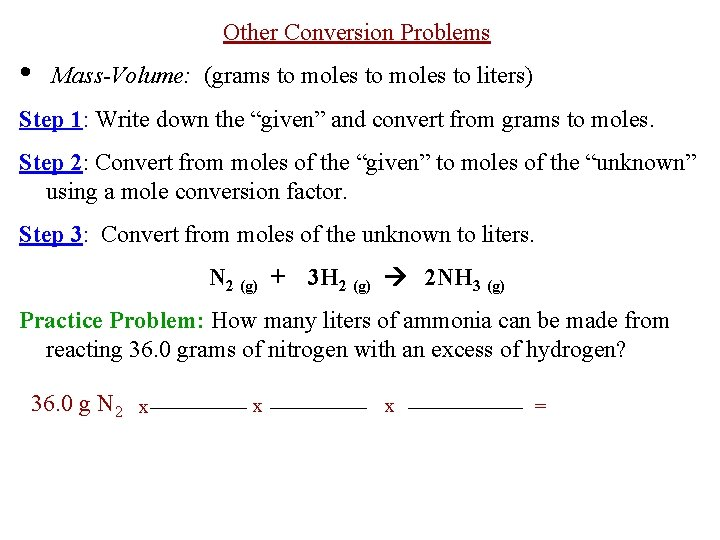Other Conversion Problems • Mass-Volume: (grams to moles to liters) Step 1: Write down the “given” and convert from grams to moles. Step 2: Convert from moles of the “given” to moles of the “unknown” using a mole conversion factor. Step 3: Convert from moles of the unknown to liters. N 2 (g) + 3 H 2 (g) 2 NH 3 (g) Practice Problem: How many liters of ammonia can be made from reacting 36. 0 grams of nitrogen with an excess of hydrogen? 36. 0 g N 2 x x x =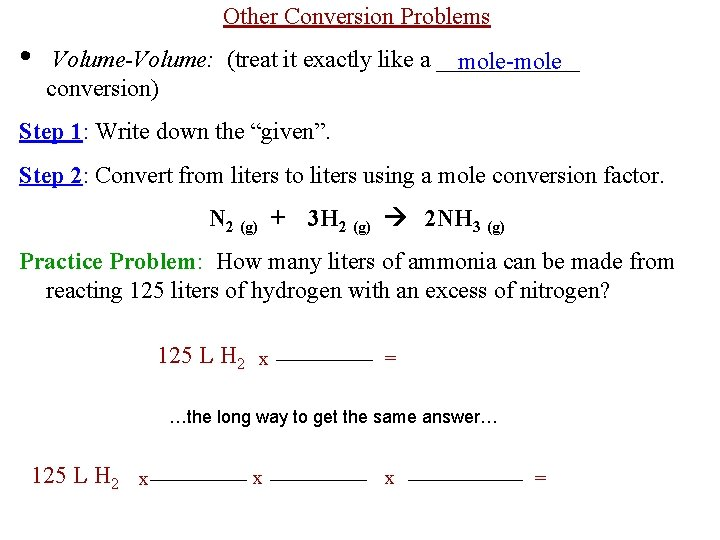Other Conversion Problems • Volume-Volume: (treat it exactly like a ______ mole-mole conversion) Step 1: Write down the “given”. Step 2: Convert from liters to liters using a mole conversion factor. N 2 (g) + 3 H 2 (g) 2 NH 3 (g) Practice Problem: How many liters of ammonia can be made from reacting 125 liters of hydrogen with an excess of nitrogen? 125 L H 2 x = …the long way to get the same answer… 125 L H 2 x x x =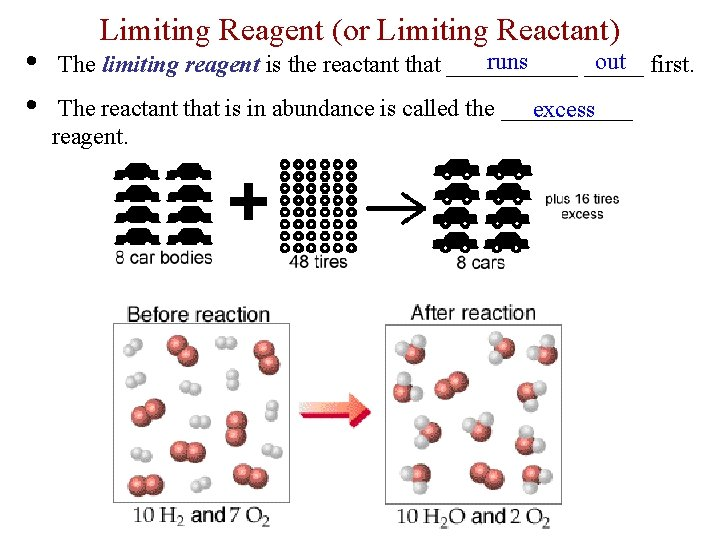• • Limiting Reagent (or Limiting Reactant) runs out first. The limiting reagent is the reactant that ______ The reactant that is in abundance is called the ______ excess reagent.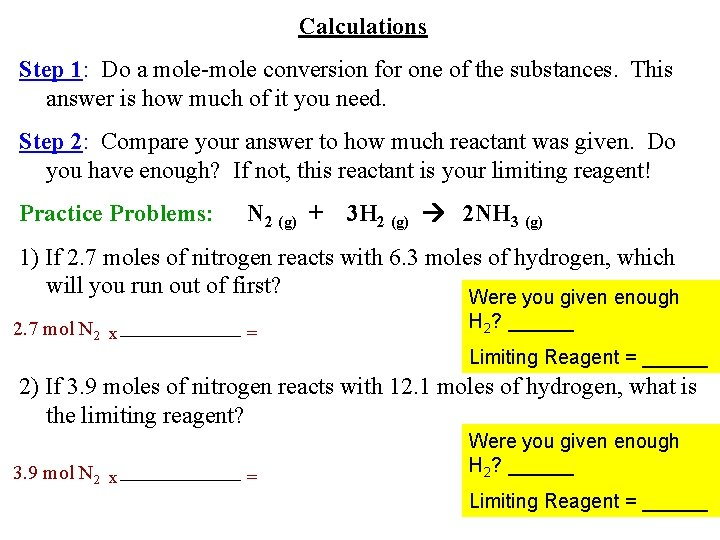Calculations Step 1: Do a mole-mole conversion for one of the substances. This answer is how much of it you need. Step 2: Compare your answer to how much reactant was given. Do you have enough? If not, this reactant is your limiting reagent! Practice Problems: N 2 (g) + 3 H 2 (g) 2 NH 3 (g) 1) If 2. 7 moles of nitrogen reacts with 6. 3 moles of hydrogen, which will you run out of first? Were you given enough 2. 7 mol N 2 x = H 2? ______ Limiting Reagent = ______ 2) If 3. 9 moles of nitrogen reacts with 12. 1 moles of hydrogen, what is the limiting reagent? 3. 9 mol N 2 x = Were you given enough H 2? ______ Limiting Reagent = ______Excess Reagent (or Excess Reactant) How many moles of excess reagent do you have? Step 1: Do a mole-mole conversion starting with the limiting reagent as the given. • The answer you get is how much of the excess reagent you need to completely react with the limiting reagent. • Sometimes you get lucky and you already did this conversion from the previous problem! Step 2: Subtract this answer from the amount given in the original problem, and that is how many moles of excess reagent there are.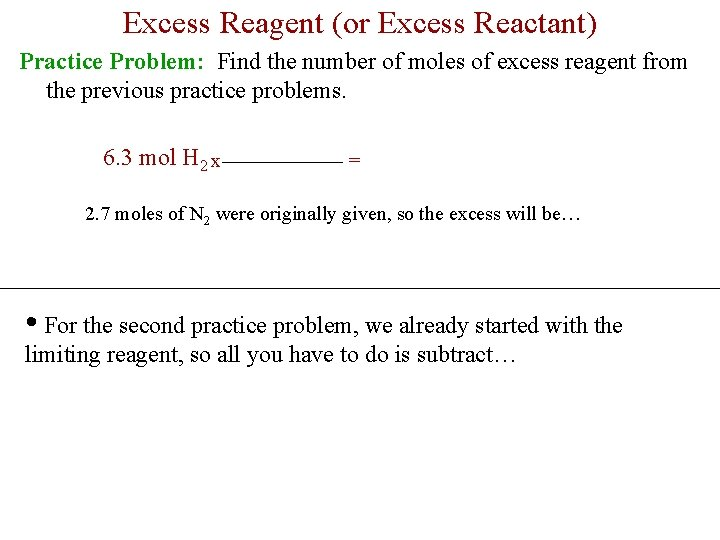Excess Reagent (or Excess Reactant) Practice Problem: Find the number of moles of excess reagent from the previous practice problems. 6. 3 mol H 2 x = 2. 7 moles of N 2 were originally given, so the excess will be… • For the second practice problem, we already started with the limiting reagent, so all you have to do is subtract…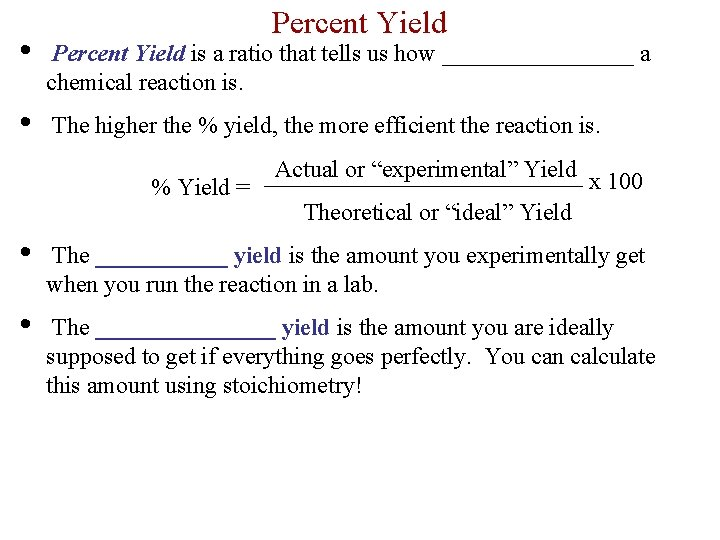Percent Yield • Percent Yield is a ratio that tells us how ________ a chemical reaction is. • The higher the % yield, the more efficient the reaction is. % Yield = Actual or “experimental” Yield x 100 Theoretical or “ideal” Yield • The ______ yield is the amount you experimentally get when you run the reaction in a lab. • The ________ yield is the amount you are ideally supposed to get if everything goes perfectly. You can calculate this amount using stoichiometry!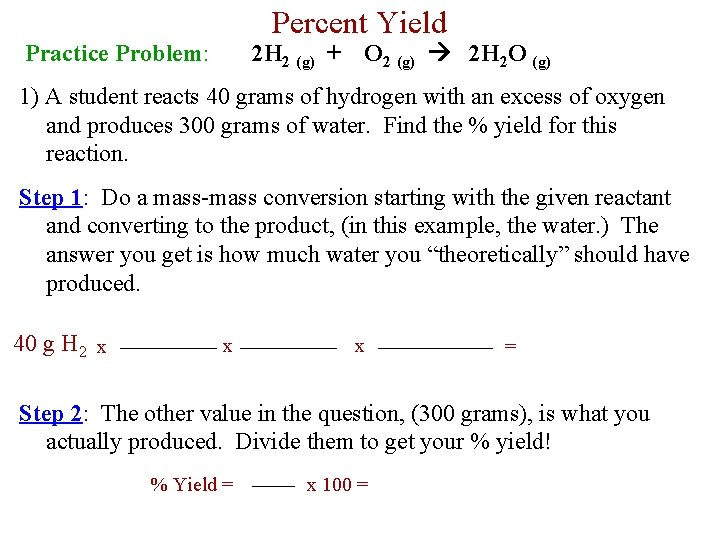Percent Yield Practice Problem: 2 H 2 (g) + O 2 (g) 2 H 2 O (g) 1) A student reacts 40 grams of hydrogen with an excess of oxygen and produces 300 grams of water. Find the % yield for this reaction. Step 1: Do a mass-mass conversion starting with the given reactant and converting to the product, (in this example, the water. ) The answer you get is how much water you “theoretically” should have produced. 40 g H 2 x x x = Step 2: The other value in the question, (300 grams), is what you actually produced. Divide them to get your % yield! % Yield = x 100 =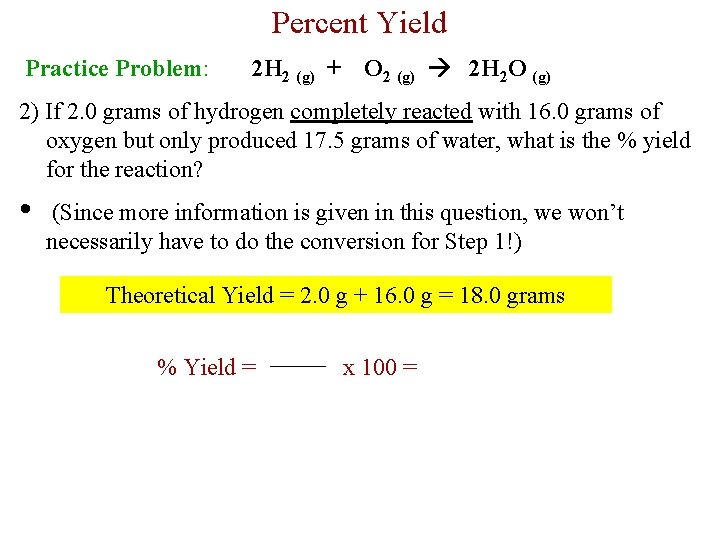Percent Yield Practice Problem: 2 H 2 (g) + O 2 (g) 2 H 2 O (g) 2) If 2. 0 grams of hydrogen completely reacted with 16. 0 grams of oxygen but only produced 17. 5 grams of water, what is the % yield for the reaction? • (Since more information is given in this question, we won’t necessarily have to do the conversion for Step 1!) Theoretical Yield = 2. 0 g + 16. 0 g = 18. 0 grams % Yield = x 100 =